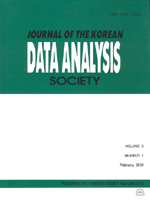상세검색
최근 검색어 전체 삭제
다국어입력
즐겨찾기0KCI등재 학술저널

# An Image Contrast Enhancement Using New Variational Framework

• 등재여부 : KCI등재
• 2014.08
• 1735 - 1743 (9 pages)

In this work, we propose new variational technique for image contrast enhancement which utilizes global and local information around each pixel. First, we have defined the energy functional by a weighted linear combination of three terms which are called on a local contrast term, a global contrast term and a dispersion term. The first one is a local contrast term that can lead to improve the contrast of an input image by increasing the grey-level differences between each pixel and its neighboring to utilize contextual information around each pixel. The second one is global contrast term, which can lead to enhance a contrast of image by minimizing the difference between its empirical distribution function and a cumulative distribution function to make the probability distribution of pixel values becoming a symmetric distribution about median. The third one is a dispersion term that control the departure between new pixel value and original pixel value of image while preserving original image characteristics as possible. Second, we derive the Euler-Lagrange equation for true image that can achieve the minimum of the proposed functional by using the fundamental lemma based on the calculus of variations. Finally, by conducting various experiments, we can demonstrate that the proposed method can enhance the contrast of images better than existing techniques.

1. Introduction

2. Proposed Method

3. Experiments

4. Conclusion

References